# Using the FAµST API in Algorithms

After the little tour we've done in the previous live scripts, about the creation of Faust objects, and their manipulation, we shall see in this third live script how the FAµST API can be deployed seamlessly in algorithms. Our example, already mentioned in the second live script, will be the Orthogonal Matching Pursuit algorithm (OMP).
This algorithm comes up in the dictionary learning problem. Assuming that the reader is already familiar with this problem we will not treat the theory behind. There is not so much to say so let's go straight to the code example.
1. The Toy OMP Algorithm Implementation
You'll find the implementation we're talking about here: tomp.mlx.
The most important point to notice in this code is that except the import part in the header, all the code seems to be a natural Matlab implementation of OMP.
This is in fact the core philosophy of the FAµST API, as explained in previous live scripts and also in the API documentation, we made sure that a Faust can be seen as a Matlab matrix. Hence this code is in fact totally compatible with the two APIs: the function argument D, which is the dictionary, can be indifferently a matfaust.Faust object or a Matlab matrix.
A secondary point is that this implementation is more like a toy concept (as indicated by the "t" in the function name). A more advanced and optimized version is introduced in the last part of this live script. In particular this version allows to define the algorithm stopping criterion according to the error tolerance the user wants.
Next we will test this implementation in both cases. But first, let us define a test case.
2. The Test Case Dictionary
For convenience, we shall set up a dictionary which guarantees uniqueness of sufficiently sparse representations. The dictionary is the concatenation of an identity matrix and a Hadamard matrix, and because we work with Faust objects, this concatenation will be a Faust object.
Below is the block matrix of our dictionary: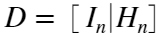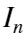is the identity and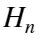the orthonormal Hadamard matrix, with n being a power of two.
The condition on which the uniqueness of the sparse representation x of a vector y is ensured is defined by the following inequality:where μ denotes the coherence of the dictionary and in the case of our specially crafted dictionary.
.
So let's construct the Faust of D, compute y for a sparse enough x and test our OMP implementation to find out if we effectively retrieve this unique x as we should according to this theorem.
Note that, for a better view and understanding you might consult this article .
n = 128;
FD = [ matfaust.eye(n), matfaust.wht(n) ];
D = full(FD)
D = 128×256
1 0 0 0 0 0 0 0 0 0 0 0 0 0 0 0 0 0 0 0 0 0 0 0 0 0 0 0 0 0 0 0 0 0 0 0 0 0 0 0 0 0 0 0 0 0 0 0 0 0 0 1 0 0 0 0 0 0 0 0 0 0 0 0 0 0 0 0 0 0 0 0 0 0 0 0 0 0 0 0 0 0 0 0 0 0 0 0 0 0 0 0 0 0 0 0 0 0 0 0 0 0 1 0 0 0 0 0 0 0 0 0 0 0 0 0 0 0 0 0 0 0 0 0 0 0 0 0 0 0 0 0 0 0 0 0 0 0 0 0 0 0 0 0 0 0 0 0 0 0 0 0 0 1 0 0 0 0 0 0 0 0 0 0 0 0 0 0 0 0 0 0 0 0 0 0 0 0 0 0 0 0 0 0 0 0 0 0 0 0 0 0 0 0 0 0 0 0 0 0 0 0 0 0 1 0 0 0 0 0 0 0 0 0 0 0 0 0 0 0 0 0 0 0 0 0 0 0 0 0 0 0 0 0 0 0 0 0 0 0 0 0 0 0 0 0 0 0 0 0 0 0 0 0 0 1 0 0 0 0 0 0 0 0 0 0 0 0 0 0 0 0 0 0 0 0 0 0 0 0 0 0 0 0 0 0 0 0 0 0 0 0 0 0 0 0 0 0 0 0 0 0 0 0 0 0 1 0 0 0 0 0 0 0 0 0 0 0 0 0 0 0 0 0 0 0 0 0 0 0 0 0 0 0 0 0 0 0 0 0 0 0 0 0 0 0 0 0 0 0 0 0 0 0 0 0 0 1 0 0 0 0 0 0 0 0 0 0 0 0 0 0 0 0 0 0 0 0 0 0 0 0 0 0 0 0 0 0 0 0 0 0 0 0 0 0 0 0 0 0 0 0 0 0 0 0 0 0 1 0 0 0 0 0 0 0 0 0 0 0 0 0 0 0 0 0 0 0 0 0 0 0 0 0 0 0 0 0 0 0 0 0 0 0 0 0 0 0 0 0 0 0 0 0 0 0 0 0 0 1 0 0 0 0 0 0 0 0 0 0 0 0 0 0 0 0 0 0 0 0 0 0 0 0 0 0 0 0 0 0 0 0 0 0 0 0 0 0 0 0
Now that we have our dictionary both defined as a Faust (FD) and as a matrix (D), let's construct our reference sparse vector x, we'll call it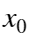.
x0 = zeros(2*n, 1);
nnz = floor(.5*(1+sqrt(n)));
nonzero_inds = randperm(2*n);
nonzero_inds = nonzero_inds(1:nnz)
nonzero_inds = 1×6
139 1 192 6 44 161
% we got nnz indices, now build the vector x0
x0(nonzero_inds, 1) = abs(randn(size(nonzero_inds, 2), 1));
disp(strcat('l0 norm of x0: ', int2str(size(nonzeros(x0), 1))))
l0 norm of x0:6
It remains to compute y.
y = D*x0;
Our test case is complete, we are fully prepared to run the OMP algorithm using a well-defined dictionary as a Faust or as numpy array, this should retrieve our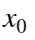from the vector y. Let's try!
3. Running the Algorithm
x = tomp(y, FD, nnz);
nonzeros(x)
ans = 2×1
0.0220 0.0220
nonzeros(x0)
ans = 6×1
0.5205 1.9929 1.6359 0.6410 0.5590 0.3807
[I, ~, ~] = find(x-x0)
I = 6×1
1 6 44 139 161 192
[I, ~, ~] = find(x)
I = 2×1
6 44
assert(all(x-x0 < 10^-6));
disp('We succeeded to retrieve x0, OMP works!')
We succeeded to retrieve x0, OMP works!
We tested OMP on a Faust, go ahead and verify what we was aiming at in the first part of the live script: is this OMP implementation really working identically on a Faust and a real array.
x = tomp(y, D, nnz);
assert(all(x-x0 < 10^-6));
disp('We succeeded to retrieve x0, OMP works!')
We succeeded to retrieve x0, OMP works!
We can conclude that the algorithm is indeed available to both Matlab array and Faust worlds, and we can imagine surely that other algorithms are reachable through the FAµST API. That's anyway in that purpose that the FAµST library will be extended if needed in the future.
4. An OMP-Cholesky Implementation
Speaking of the OMP algorithm and the possibility to implement other optimization algorithms with FAµST, it would be a pity not to mention that the library is delivered with another implementation of OMP.
This implementation is actually an optimized version which takes advantage of the Cholesky factorization to simplify the least-square problem to solve at each iteration. This algorithm is implemented into the tools module of matfaust.
import matfaust.tools.omp
help matfaust.rand
========================================================================================== > @brief Generates a random Faust. > > @warning if this function is imported through 'import matfaust.rand' or 'import matfaust.*' the Matlab builtin function rand will be unreachable (matfaust.rand will be called instead). So, generally, it is not advisable to import this function, rather directly call matfaust.rand without any import. > > @b Usage > > &nbsp;&nbsp;&nbsp; @b rand(@b M,@b N) generates a M-by-N Faust object. The numbers of rows and columns of intermediary factors are all randomly chosen between M and N (included). The number of factors is 5. The factors are sparse and real. The nnz per row of factors is 5. > > &nbsp;&nbsp;&nbsp; @b rand(@b M, @b N, @b 'num_factors', @b NF, @b 'dim_sizes', @b S) with NF and S two integers, generates a M-by-N Faust of NF factors. The factor size is S for both dimensions (except the first factor number of rows and the last factor number of columns which are respectively M and N). The factors are sparse and real. > > &nbsp;&nbsp;&nbsp; @b rand(@b M, @b N, @b 'num_factors', [@b N1, @b N2], @b 'dim_sizes', @b S) same as above except that here the number of factors is randomly chosen between N1 and N2 inclusively. > > &nbsp;&nbsp;&nbsp; @b rand(@b M, @b N, @b 'num_factors', [@b N1, N@b 2], [@b S1, @b S2]) or @b rand(@b M, N@b , @b 'num_factors', @b NF, @b 'dim_sizes', [@b S1, @b S2]) same as above except that here the intermediary factor dimension sizes are random; the number of rows and columns are both randomly chosen between S1 and S2 inclusively. > > &nbsp;&nbsp;&nbsp; @b rand(@b M, @b N, @b 'num_factors', @b NF, @b 'dim_sizes', @b S, @b 'density', @b D) or @b rand(@b M, @b N, @b 'num_factors', [@b N1, @b N2], @b 'dim_sizes', [@b S1, @b S2], @b 'density', @b D) same as above but specifying D the approximate density of each factor. > > &nbsp;&nbsp;&nbsp; @b rand(@b M, @b N, @b 'num_factors', @b NF, @b 'dim_sizes', @b S, @b 'density', @b D, @b 'per_row', @b true) or @b rand(@b M, @b N, @b 'num_factors', [@b N1, @b N2], @b 'dim_sizes', [@b S1, @b S2], @b 'density',@b D, @b 'per_row', true) same as above but specifying D, the density of each factor per row ('per_row', true) or per column ('per_row', false). > > &nbsp;&nbsp;&nbsp; @b @b rand(@b M, @b N, @b 'num_factors', @b NF, @b 'dim_sizes', @b S, @b 'density', @b D, @b 'fac_type', @b 'dense') or @b rand(@b M, @b N, @b 'num_factors', @b [@b N1, @b N2], @b 'dim_sizes', [@b S1, @b S2], @b 'density', @b D, @b 'fac_type', @b 'dense') same as above but generating only dense matrices as factors. > > &nbsp;&nbsp;&nbsp; @b rand(@b M, @b N, @b 'num_factors', @b NF, @b 'dim_sizes', @b S, @b 'density', @b D, @b 'mixed') or @b rand(@b M, @b N, @b 'num_factors', [@b N1, @b N2], @b 'dim_sizes', [@b S1, @b S2], @b 'density', @b D, @b 'fac_type', @b 'sparse') same as above but generating either sparse or dense matrices as factors. > > &nbsp;&nbsp;&nbsp; @b rand(@b M, @b N, @b 'num_factors', @b NF, @b 'dim_sizes', @b S, @b 'density', @b D, @b 'fac_type', @b 'sparse', @b 'field' , @b 'complex'), @b rand(@b M, @b N, @b 'num_factors', [@b N1, @b N2], @b 'dim_sizes', [@b S1, @b S2], @b 'density', @b D, @b 'fac_type', @b 'sparse', @b 'per_row', @b false), rand(@b M, @b N, @b 'num_factors', @b NF, @b 'dim_sizes', @b S, @b 'density', @b D, @b 'fac_type', @b 'dense', @b 'field' , @b 'complex') or @b rand(@b M, @b N, @b 'num_factors', [@b N1, @b N2], @b 'dim_sizes', [@b S1, @b S2], @b D, @b 'fac_type', @b 'dense', @b 'field', @b 'complex') same as above but generating a complex Faust, that is, matrices defined over the complex field. > > > > > > > > @param M the Faust number of rows. > @param N the Faust number of columns. > @param 'num_factors', NF if it's an integer it is the number of random factors to set in the Faust. > If NF is a vector of 2 integers then the > number of factors is set randomly between > NF(1) and NF(2) (inclusively). Defaultly, a 5 factors long Faust is generated. > @param 'dim_sizes',S if it's an integer all Faust factors are square matrices (except maybe the first > and last ones, depending on M and N). The size of the intermediary square factors is S**2. > If it's a vector of 2 integers then the number of rows and columns are both a random number between size_dims(1) and > size_dims(2) (inclusively). Defaultly, dim_sizes is [M, N]. > @param 'density',D the approximate density of generated factors. > D must be a floating point number greater than 0 and lower of equal to 1. > The default value is such that each factor gets 5 non-zero > elements per row, if per_row is true, or per column otherwise. > A density of zero is equivalent to the default case. > @param 'per_row',bool this argument is to specify the density per row or per column. > By default the density is set per row and is such that the Faust's factors will have 5 non-zero elements per row. > @param fac_type, str the storage representation of factors. str must be 'sparse', 'dense' or 'mixed'. > The latter designates a mix of dense and sparse matrices in the generated Faust (the choice is made according > to a uniform distribution). > The default value is 'sparse'. > @param 'field', str str is either 'real' or 'complex' (the Faust field). > The default value is 'real'. > @param 'dev', str 'gpu or 'cpu' to create the random Faust on CPU or GPU (by default on CPU). > @param 'class', str 'double' (by default) or 'single' to select the scalar type used for the Faust generated. > > > @retval F the random Faust. > > @b Example @b 1 > @code > >> F = matfaust.rand(10,5) > > F = > > Faust size 10x5, density 4.32, nnz_sum 216, 5 factor(s): > - FACTOR 0 (real) SPARSE, size 10x9, density 0.555556, nnz 50 > - FACTOR 1 (real) SPARSE, size 9x6, density 0.666667, nnz 36 > - FACTOR 2 (real) SPARSE, size 6x10, density 0.5, nnz 30 > - FACTOR 3 (real) SPARSE, size 10x10, density 0.5, nnz 50 > - FACTOR 4 (real) SPARSE, size 10x5, density 1, nnz 50 > @endcode > > @b Example @b 2 > @code > % in a matlab terminal > >> G = matfaust.rand(10, 10, 'num_factors', 2, 'dim_sizes', 10, 'density', .5, 'mixed', 'complex') > > G = > > Faust size 10x10, density 1, nnz_sum 100, 2 factor(s): > - FACTOR 0 (complex) SPARSE, size 10x10, density 0.5, nnz 50 > - FACTOR 1 (complex) DENSE, size 10x10, density 0.5, nnz 50 > @endcode > > @b Example @b 3 > @code > >> H = matfaust.rand(10, 10, 'num_factors', [2, 5], 'dim_sizes', [10, 20], 'density', .5, 'fac_type', 'dense') > > H = > > Faust size 5x10, density 4.64, nnz_sum 232, 3 factor(s): > - FACTOR 0 (real) DENSE, size 5x14, density 0.5, nnz 35 > - FACTOR 1 (real) DENSE, size 14x17, density 0.470588, nnz 112 > - FACTOR 2 (real) DENSE, size 17x10, density 0.5, nnz 85 > > @endcode > > <p>@b See @b also Faust.Faust, matfaust.rand_bsr. ==========================================================================================
This implementation is integrated into matfaust as a tool for the Brain Source Localization (BSL) demo which is documented here.
To show off a little, let's run this demo.
Warning: the demo takes a few minutes.
matfaust.demo.bsl.BSL()
Stopping. Exact signal representation found! Stopping. Exact signal representation found! Stopping. Exact signal representation found! Stopping. Exact signal representation found! Stopping. Exact signal representation found! Stopping. Exact signal representation found! Stopping. Exact signal representation found! Stopping. Exact signal representation found! Stopping. Exact signal representation found! Stopping. Exact signal representation found! Stopping. Exact signal representation found! Stopping. Exact signal representation found! Stopping. Exact signal representation found! Stopping. Exact signal representation found! Stopping. Exact signal representation found! Stopping. Exact signal representation found! Stopping. Exact signal representation found! Stopping. Exact signal representation found! Stopping. Exact signal representation found! Stopping. Exact signal representation found! Stopping. Exact signal representation found! Stopping. Exact signal representation found! Stopping. Exact signal representation found! Stopping. Exact signal representation found! Stopping. Exact signal representation found! Stopping. Exact signal representation found! Stopping. Exact signal representation found! Stopping. Exact signal representation found! Stopping. Exact signal representation found! Stopping. Exact signal representation found! Stopping. Exact signal representation found! Stopping. Exact signal representation found! Stopping. Exact signal representation found! Stopping. Exact signal representation found! Stopping. Exact signal representation found! Stopping. Exact signal representation found! Stopping. Exact signal representation found! Stopping. Exact signal representation found! Stopping. Exact signal representation found! Stopping. Exact signal representation found! Stopping. Exact signal representation found! Stopping. Exact signal representation found! Stopping. Exact signal representation found! Stopping. Exact signal representation found! Stopping. Exact signal representation found! Stopping. Exact signal representation found! Stopping. Exact signal representation found! Stopping. Exact signal representation found! Stopping. Exact signal representation found! Stopping. Exact signal representation found! Stopping. Exact signal representation found! Stopping. Exact signal representation found! Stopping. Exact signal representation found! Stopping. Exact signal representation found! Stopping. Exact signal representation found! Stopping. Exact signal representation found! Stopping. Exact signal representation found! Stopping. Exact signal representation found! Stopping. Exact signal representation found! Stopping. Exact signal representation found! Stopping. Exact signal representation found! Stopping. Exact signal representation found! Stopping. Exact signal representation found! Stopping. Exact signal representation found! Stopping. Exact signal representation found! Stopping. Exact signal representation found! Stopping. Exact signal representation found! Stopping. Exact signal representation found! Stopping. Exact signal representation found! Stopping. Exact signal representation found! Stopping. Exact signal representation found! Stopping. Exact signal representation found! Stopping. Exact signal representation found! Stopping. Exact signal representation found! Stopping. Exact signal representation found! Stopping. Exact signal representation found! Stopping. Exact signal representation found! Stopping. Exact signal representation found! Stopping. Exact signal representation found! Stopping. Exact signal representation found! Stopping. Exact signal representation found! Stopping. Exact signal representation found! Stopping. Exact signal representation found! Stopping. Exact signal representation found! Stopping. Exact signal representation found! Stopping. Exact signal representation found! Stopping. Exact signal representation found! Stopping. Exact signal representation found! Stopping. Exact signal representation found! Stopping. Exact signal representation found! Stopping. Exact signal representation found! Stopping. Exact signal representation found! Stopping. Exact signal representation found! Stopping. Exact signal representation found! Stopping. Exact signal representation found! Stopping. Exact signal representation found! Stopping. Exact signal representation found! Stopping. Exact signal representation found! Stopping. Exact signal representation found! Stopping. Exact signal representation found! Stopping. Exact signal representation found! Stopping. Exact signal representation found! Stopping. Exact signal representation found! Stopping. Exact signal representation found! Stopping. Exact signal representation found! Stopping. Exact signal representation found! Stopping. Exact signal representation found! Stopping. Exact signal representation found! Stopping. Exact signal representation found! Stopping. Exact signal representation found! Stopping. Exact signal representation found! Stopping. Exact signal representation found! Stopping. Exact signal representation found! Stopping. Exact signal representation found! Stopping. Exact signal representation found! Stopping. Exact signal representation found! Stopping. Exact signal representation found! Stopping. Exact signal representation found! Stopping. Exact signal representation found! Stopping. Exact signal representation found! Stopping. Exact signal representation found! Stopping. Exact signal representation found! Stopping. Exact signal representation found! Stopping. Exact signal representation found! Stopping. Exact signal representation found! Stopping. Exact signal representation found! Stopping. Exact signal representation found! Stopping. Exact signal representation found! Stopping. Exact signal representation found! Stopping. Exact signal representation found! Stopping. Exact signal representation found! Stopping. Exact signal representation found! Stopping. Exact signal representation found! Stopping. Exact signal representation found! Stopping. Exact signal representation found! Stopping. Exact signal representation found! Stopping. Exact signal representation found! Stopping. Exact signal representation found! Stopping. Exact signal representation found! Stopping. Exact signal representation found! Stopping. Exact signal representation found! Stopping. Exact signal representation found! Stopping. Exact signal representation found! Stopping. Exact signal representation found! Stopping. Exact signal representation found! Stopping. Exact signal representation found! Stopping. Exact signal representation found! Stopping. Exact signal representation found! Stopping. Exact signal representation found! Stopping. Exact signal representation found! Stopping. Exact signal representation found! Stopping. Exact signal representation found! Stopping. Exact signal representation found! Stopping. Exact signal representation found! Stopping. Exact signal representation found! Stopping. Exact signal representation found! Stopping. Exact signal representation found! Stopping. Exact signal representation found! Stopping. Exact signal representation found! Stopping. Exact signal representation found! Stopping. Exact signal representation found! Stopping. Exact signal representation found! Stopping. Exact signal representation found! Stopping. Exact signal representation found! Stopping. Exact signal representation found! Stopping. Exact signal representation found! Stopping. Exact signal representation found! Stopping. Exact signal representation found! Stopping. Exact signal representation found! Stopping. Exact signal representation found! Stopping. Exact signal representation found! Stopping. Exact signal representation found! Stopping. Exact signal representation found! Stopping. Exact signal representation found! Stopping. Exact signal representation found! Stopping. Exact signal representation found! Stopping. Exact signal representation found! Stopping. Exact signal representation found! Stopping. Exact signal representation found! Stopping. Exact signal representation found! Stopping. Exact signal representation found! Stopping. Exact signal representation found! Stopping. Exact signal representation found! Stopping. Exact signal representation found! Stopping. Exact signal representation found! Stopping. Exact signal representation found! Stopping. Exact signal representation found! Stopping. Exact signal representation found! Stopping. Exact signal representation found! Stopping. Exact signal representation found! Stopping. Exact signal representation found! Stopping. Exact signal representation found! Stopping. Exact signal representation found! Stopping. Exact signal representation found! Stopping. Exact signal representation found! Stopping. Exact signal representation found! Stopping. Exact signal representation found! Stopping. Exact signal representation found! Stopping. Exact signal representation found! Stopping. Exact signal representation found! Stopping. Exact signal representation found! Stopping. Exact signal representation found! Stopping. Exact signal representation found! Stopping. Exact signal representation found! Stopping. Exact signal representation found! Stopping. Exact signal representation found! Stopping. Exact signal representation found! Stopping. Exact signal representation found! Stopping. Exact signal representation found! Stopping. Exact signal representation found! Stopping. Exact signal representation found! Stopping. Exact signal representation found! Stopping. Exact signal representation found! Stopping. Exact signal representation found! Stopping. Exact signal representation found! Stopping. Exact signal representation found! Stopping. Exact signal representation found! Stopping. Exact signal representation found! Stopping. Exact signal representation found! Stopping. Exact signal representation found! Stopping. Exact signal representation found! Stopping. Exact signal representation found! Stopping. Exact signal representation found! Stopping. Exact signal representation found! Stopping. Exact signal representation found! Stopping. Exact signal representation found! Stopping. Exact signal representation found! Stopping. Exact signal representation found! Stopping. Exact signal representation found! Stopping. Exact signal representation found! Stopping. Exact signal representation found! Stopping. Exact signal representation found! Stopping. Exact signal representation found! Stopping. Exact signal representation found! Stopping. Exact signal representation found! Stopping. Exact signal representation found! Stopping. Exact signal representation found! Stopping. Exact signal representation found! Stopping. Exact signal representation found! Stopping. Exact signal representation found! Stopping. Exact signal representation found! Stopping. Exact signal representation found! Stopping. Exact signal representation found! Stopping. Exact signal representation found! Stopping. Exact signal representation found! Stopping. Exact signal representation found! Stopping. Exact signal representation found! Stopping. Exact signal representation found! Stopping. Exact signal representation found! Stopping. Exact signal representation found! Stopping. Exact signal representation found! Stopping. Exact signal representation found! Stopping. Exact signal representation found! Stopping. Exact signal representation found! Stopping. Exact signal representation found! Stopping. Exact signal representation found! Stopping. Exact signal representation found! Stopping. Exact signal representation found! Stopping. Exact signal representation found! Stopping. Exact signal representation found! Stopping. Exact signal representation found! Stopping. Exact signal representation found! Stopping. Exact signal representation found! Stopping. Exact signal representation found! Stopping. Exact signal representation found! Stopping. Exact signal representation found! Stopping. Exact signal representation found! Stopping. Exact signal representation found! Stopping. Exact signal representation found! Stopping. Exact signal representation found! Stopping. Exact signal representation found! Stopping. Exact signal representation found! Stopping. Exact signal representation found! Stopping. Exact signal representation found! Stopping. Exact signal representation found! Stopping. Exact signal representation found! Stopping. Exact signal representation found! Stopping. Exact signal representation found! Stopping. Exact signal representation found! Stopping. Exact signal representation found! Stopping. Exact signal representation found! Stopping. Exact signal representation found! Stopping. Exact signal representation found! Stopping. Exact signal representation found! Stopping. Exact signal representation found! Stopping. Exact signal representation found! Stopping. Exact signal representation found! Stopping. Exact signal representation found! Stopping. Exact signal representation found! Stopping. Exact signal representation found! Stopping. Exact signal representation found! Stopping. Exact signal representation found! Stopping. Exact signal representation found! Stopping. Exact signal representation found! Stopping. Exact signal representation found! Stopping. Exact signal representation found! Stopping. Exact signal representation found! Stopping. Exact signal representation found! Stopping. Exact signal representation found! Stopping. Exact signal representation found! Stopping. Exact signal representation found! Stopping. Exact signal representation found! Stopping. Exact signal representation found! Stopping. Exact signal representation found! Stopping. Exact signal representation found! Stopping. Exact signal representation found! Stopping. Exact signal representation found! Stopping. Exact signal representation found! Stopping. Exact signal representation found! Stopping. Exact signal representation found! Stopping. Exact signal representation found! Stopping. Exact signal representation found! Stopping. Exact signal representation found! Stopping. Exact signal representation found! Stopping. Exact signal representation found! Stopping. Exact signal representation found! Stopping. Exact signal representation found! Stopping. Exact signal representation found! Stopping. Exact signal representation found! Stopping. Exact signal representation found! Stopping. Exact signal representation found! Stopping. Exact signal representation found! Stopping. Exact signal representation found! Stopping. Exact signal representation found! Stopping. Exact signal representation found! Stopping. Exact signal representation found! Stopping. Exact signal representation found! Stopping. Exact signal representation found! Stopping. Exact signal representation found! Stopping. Exact signal representation found! Stopping. Exact signal representation found! Stopping. Exact signal representation found! Stopping. Exact signal representation found! Stopping. Exact signal representation found! Stopping. Exact signal representation found! Stopping. Exact signal representation found! Stopping. Exact signal representation found! Stopping. Exact signal representation found! Stopping. Exact signal representation found! Stopping. Exact signal representation found! Stopping. Exact signal representation found! Stopping. Exact signal representation found! Stopping. Exact signal representation found! Stopping. Exact signal representation found! Stopping. Exact signal representation found! Stopping. Exact signal representation found! Stopping. Exact signal representation found! Stopping. Exact signal representation found! Stopping. Exact signal representation found! Stopping. Exact signal representation found! Stopping. Exact signal representation found! Stopping. Exact signal representation found! Stopping. Exact signal representation found! Stopping. Exact signal representation found! Stopping. Exact signal representation found! Stopping. Exact signal representation found! Stopping. Exact signal representation found! Stopping. Exact signal representation found! Stopping. Exact signal representation found! Stopping. Exact signal representation found! Stopping. Exact signal representation found! Stopping. Exact signal representation found! Stopping. Exact signal representation found! Stopping. Exact signal representation found! Stopping. Exact signal representation found! Stopping. Exact signal representation found! Stopping. Exact signal representation found! Stopping. Exact signal representation found! Stopping. Exact signal representation found! Stopping. Exact signal representation found! Stopping. Exact signal representation found! Stopping. Exact signal representation found! Stopping. Exact signal representation found! Stopping. Exact signal representation found! Stopping. Exact signal representation found! Stopping. Exact signal representation found! Stopping. Exact signal representation found! Stopping. Exact signal representation found! Stopping. Exact signal representation found! Stopping. Exact signal representation found! Stopping. Exact signal representation found! Stopping. Exact signal representation found! Stopping. Exact signal representation found! Stopping. Exact signal representation found! Stopping. Exact signal representation found! Stopping. Exact signal representation found! Stopping. Exact signal representation found! Stopping. Exact signal representation found! Stopping. Exact signal representation found! Stopping. Exact signal representation found! Stopping. Exact signal representation found! Stopping. Exact signal representation found! Stopping. Exact signal representation found! Stopping. Exact signal representation found! Stopping. Exact signal representation found! Stopping. Exact signal representation found! Stopping. Exact signal representation found! Stopping. Exact signal representation found! Stopping. Exact signal representation found! Stopping. Exact signal representation found! Stopping. Exact signal representation found! Stopping. Exact signal representation found! Stopping. Exact signal representation found! Stopping. Exact signal representation found! Stopping. Exact signal representation found! Stopping. Exact signal representation found! Stopping. Exact signal representation found! Stopping. Exact signal representation found! Stopping. Exact signal representation found! Stopping. Exact signal representation found! Stopping. Exact signal representation found! Stopping. Exact signal representation found! Stopping. Exact signal representation found! Stopping. Exact signal representation found! Stopping. Exact signal representation found! Stopping. Exact signal representation found! Stopping. Exact signal representation found! Stopping. Exact signal representation found! Stopping. Exact signal representation found! Stopping. Exact signal representation found! Stopping. Exact signal representation found! Stopping. Exact signal representation found! Stopping. Exact signal representation found! Stopping. Exact signal representation found! Stopping. Exact signal representation found! Stopping. Exact signal representation found! Stopping. Exact signal representation found! Stopping. Exact signal representation found! Stopping. Exact signal representation found! Stopping. Exact signal representation found! Stopping. Exact signal representation found! Stopping. Exact signal representation found! Stopping. Exact signal representation found! Stopping. Exact signal representation found! Stopping. Exact signal representation found! Stopping. Exact signal representation found! Stopping. Exact signal representation found! Stopping. Exact signal representation found! Stopping. Exact signal representation found! Stopping. Exact signal representation found! Stopping. Exact signal representation found! Stopping. Exact signal representation found! Stopping. Exact signal representation found! Stopping. Exact signal representation found! Stopping. Exact signal representation found! Stopping. Exact signal representation found! Stopping. Exact signal representation found! Stopping. Exact signal representation found! Stopping. Exact signal representation found! Stopping. Exact signal representation found! Stopping. Exact signal representation found! Stopping. Exact signal representation found! Stopping. Exact signal representation found! Stopping. Exact signal representation found! Stopping. Exact signal representation found! Stopping. Exact signal representation found! Stopping. Exact signal representation found! Stopping. Exact signal representation found! Stopping. Exact signal representation found! Stopping. Exact signal representation found! Stopping. Exact signal representation found! Stopping. Exact signal representation found! Stopping. Exact signal representation found! Stopping. Exact signal representation found! Stopping. Exact signal representation found! Stopping. Exact signal representation found! Stopping. Exact signal representation found! Stopping. Exact signal representation found! Stopping. Exact signal representation found! Stopping. Exact signal representation found! Stopping. Exact signal representation found! Stopping. Exact signal representation found! Stopping. Exact signal representation found! Stopping. Exact signal representation found! Stopping. Exact signal representation found! Stopping. Exact signal representation found! Stopping. Exact signal representation found! Stopping. Exact signal representation found! Stopping. Exact signal representation found! Stopping. Exact signal representation found! Stopping. Exact signal representation found! Stopping. Exact signal representation found! Stopping. Exact signal representation found! Stopping. Exact signal representation found! Stopping. Exact signal representation found! Stopping. Exact signal representation found! Stopping. Exact signal representation found! Stopping. Exact signal representation found! Stopping. Exact signal representation found! Stopping. Exact signal representation found! Stopping. Exact signal representation found! Stopping. Exact signal representation found! Stopping. Exact signal representation found! Stopping. Exact signal representation found! Stopping. Exact signal representation found! Stopping. Exact signal representation found! Stopping. Exact signal representation found! Stopping. Exact signal representation found! Stopping. Exact signal representation found! Stopping. Exact signal representation found! Stopping. Exact signal representation found! Stopping. Exact signal representation found! Stopping. Exact signal representation found! Stopping. Exact signal representation found! Stopping. Exact signal representation found! Stopping. Exact signal representation found! Stopping. Exact signal representation found! Stopping. Exact signal representation found! Stopping. Exact signal representation found! Stopping. Exact signal representation found! Stopping. Exact signal representation found! Stopping. Exact signal representation found! Stopping. Exact signal representation found! Stopping. Exact signal representation found! Stopping. Exact signal representation found! Stopping. Exact signal representation found! Stopping. Exact signal representation found! Stopping. Exact signal representation found! Stopping. Exact signal representation found! Stopping. Exact signal representation found! Stopping. Exact signal representation found! Stopping. Exact signal representation found! Stopping. Exact signal representation found! Stopping. Exact signal representation found! Stopping. Exact signal representation found! Stopping. Exact signal representation found! Stopping. Exact signal representation found! Stopping. Exact signal representation found! Stopping. Exact signal representation found! Stopping. Exact signal representation found! Stopping. Exact signal representation found! Stopping. Exact signal representation found! Stopping. Exact signal representation found! Stopping. Exact signal representation found! Stopping. Exact signal representation found! Stopping. Exact signal representation found! Stopping. Exact signal representation found! Stopping. Exact signal representation found! Stopping. Exact signal representation found! Stopping. Exact signal representation found! Stopping. Exact signal representation found! Stopping. Exact signal representation found! Stopping. Exact signal representation found! Stopping. Exact signal representation found! Stopping. Exact signal representation found! Stopping. Exact signal representation found! Stopping. Exact signal representation found! Stopping. Exact signal representation found! Stopping. Exact signal representation found! Stopping. Exact signal representation found! Stopping. Exact signal representation found! Stopping. Exact signal representation found! Stopping. Exact signal representation found! Stopping. Exact signal representation found! Stopping. Exact signal representation found! Stopping. Exact signal representation found! Stopping. Exact signal representation found! Stopping. Exact signal representation found! Stopping. Exact signal representation found! Stopping. Exact signal representation found! Stopping. Exact signal representation found! Stopping. Exact signal representation found! Stopping. Exact signal representation found! Stopping. Exact signal representation found! Stopping. Exact signal representation found! Stopping. Exact signal representation found! Stopping. Exact signal representation found! Stopping. Exact signal representation found! Stopping. Exact signal representation found! Stopping. Exact signal representation found! Stopping. Exact signal representation found! Stopping. Exact signal representation found! Stopping. Exact signal representation found! Stopping. Exact signal representation found! Stopping. Exact signal representation found! Stopping. Exact signal representation found! Stopping. Exact signal representation found! Stopping. Exact signal representation found! Stopping. Exact signal representation found! Stopping. Exact signal representation found! Stopping. Exact signal representation found! Stopping. Exact signal representation found! Stopping. Exact signal representation found! Stopping. Exact signal representation found! Stopping. Exact signal representation found! Stopping. Exact signal representation found! Stopping. Exact signal representation found! Stopping. Exact signal representation found! Stopping. Exact signal representation found! Stopping. Exact signal representation found! Stopping. Exact signal representation found! Stopping. Exact signal representation found! Stopping. Exact signal representation found! Stopping. Exact signal representation found! Stopping. Exact signal representation found! Stopping. Exact signal representation found! Stopping. Exact signal representation found! Stopping. Exact signal representation found! Stopping. Exact signal representation found! Stopping. Exact signal representation found! Stopping. Exact signal representation found! Stopping. Exact signal representation found! Stopping. Exact signal representation found! Stopping. Exact signal representation found! Stopping. Exact signal representation found! Stopping. Exact signal representation found! Stopping. Exact signal representation found! Stopping. Exact signal representation found! Stopping. Exact signal representation found! Stopping. Exact signal representation found! Stopping. Exact signal representation found! Stopping. Exact signal representation found! Stopping. Exact signal representation found! Stopping. Exact signal representation found! Stopping. Exact signal representation found! Stopping. Exact signal representation found! Stopping. Exact signal representation found! Stopping. Exact signal representation found! Stopping. Exact signal representation found! Stopping. Exact signal representation found! Stopping. Exact signal representation found! Stopping. Exact signal representation found! Stopping. Exact signal representation found! Stopping. Exact signal representation found! Stopping. Exact signal representation found! Stopping. Exact signal representation found! Stopping. Exact signal representation found! Stopping. Exact signal representation found! Stopping. Exact signal representation found! Stopping. Exact signal representation found! Stopping. Exact signal representation found! Stopping. Exact signal representation found! Stopping. Exact signal representation found! Stopping. Exact signal representation found! Stopping. Exact signal representation found! Stopping. Exact signal representation found! Stopping. Exact signal representation found! Stopping. Exact signal representation found! Stopping. Exact signal representation found! Stopping. Exact signal representation found! Stopping. Exact signal representation found! Stopping. Exact signal representation found! Stopping. Exact signal representation found! Stopping. Exact signal representation found! Stopping. Exact signal representation found! Stopping. Exact signal representation found! Stopping. Exact signal representation found! Stopping. Exact signal representation found! Stopping. Exact signal representation found! Stopping. Exact signal representation found! Stopping. Exact signal representation found! Stopping. Exact signal representation found! Stopping. Exact signal representation found! Stopping. Exact signal representation found! Stopping. Exact signal representation found! Stopping. Exact signal representation found! Stopping. Exact signal representation found! Stopping. Exact signal representation found! Stopping. Exact signal representation found! Stopping. Exact signal representation found! Stopping. Exact signal representation found! Stopping. Exact signal representation found! Stopping. Exact signal representation found! Stopping. Exact signal representation found! Stopping. Exact signal representation found! Stopping. Exact signal representation found! Stopping. Exact signal representation found! Stopping. Exact signal representation found! Stopping. Exact signal representation found! Stopping. Exact signal representation found! Stopping. Exact signal representation found! Stopping. Exact signal representation found! Stopping. Exact signal representation found! Stopping. Exact signal representation found! Stopping. Exact signal representation found! Stopping. Exact signal representation found! Stopping. Exact signal representation found! Stopping. Exact signal representation found! Stopping. Exact signal representation found! Stopping. Exact signal representation found! Stopping. Exact signal representation found! Stopping. Exact signal representation found! Stopping. Exact signal representation found! Stopping. Exact signal representation found! Stopping. Exact signal representation found! Stopping. Exact signal representation found! Stopping. Exact signal representation found! Stopping. Exact signal representation found! Stopping. Exact signal representation found! Stopping. Exact signal representation found! Stopping. Exact signal representation found! Stopping. Exact signal representation found! Stopping. Exact signal representation found! Stopping. Exact signal representation found! Stopping. Exact signal representation found! Stopping. Exact signal representation found! Stopping. Exact signal representation found! Stopping. Exact signal representation found! Stopping. Exact signal representation found! Stopping. Exact signal representation found! Stopping. Exact signal representation found! Stopping. Exact signal representation found! Stopping. Exact signal representation found! Stopping. Exact signal representation found!
matfaust.demo.bsl.Fig_BSL()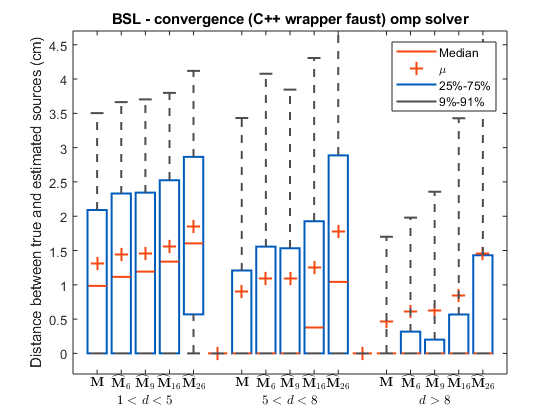Warning: Ignoring extra legend entries.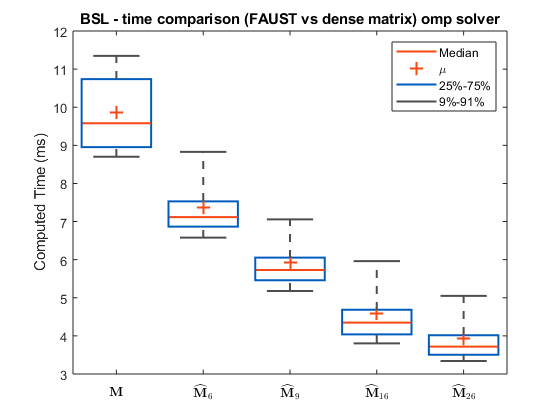Warning: Ignoring extra legend entries.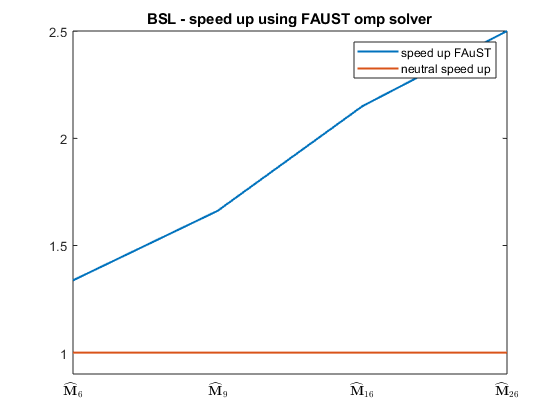**** MEG with OMP solver time comparison **** M tps : 9.8574 M_6 tps : 7.372, speed-up : 1.3371 M_9 tps : 5.9321, speed-up : 1.6617 M_16 tps : 4.5849, speed-up : 2.15 M_26 tps : 3.9414, speed-up : 2.501
What we see in this figure is that it takes a few dozens of milliseconds (the median time) to compute the BSL experiment on the dense matrix M. This is well above the time it takes with Faust approximates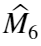to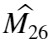in which the numbers 6 and 26 denote the Faust RCG. The greater the RCG the better the computation time is, as we already saw in the live script about Faust manipulations.
As a complementary test, let's verify that the two runs of omp() on FD and D we constructed before for the toy OMP give the same results even if the vector to retrieve is not very sparse. Here for instance,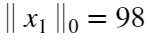.
import matfaust.tools.omp
nnz = 98;
x1 = zeros(2*n, 1);
nnz_inds = randperm(2*n);
nnz_inds = nnz_inds(1:nnz);
x1(nnz_inds, 1) = randn(size(nnz_inds,2),1);
y = FD*x1;
x2 = omp(y, D, 'maxiter', nnz);
x3 = omp(y, FD, 'maxiter', nnz);
disp(['Are x2 and x3 solutions almost equal? ' int2str(norm(x2-x3)/norm(x3) < 10^-12) ])
Are x2 and x3 solutions almost equal? 1
disp(['Is x1 retrieved into x2? ' int2str(all(x1-x2 < 10^-6)) ])
Is x1 retrieved into x2? 0
disp(['Is x1 retrieved into x3? ' int2str(all(x1-x3 < 10^-6)) ])
Is x1 retrieved into x3? 0
As expected, we didn't retrieve our starting x1 (the reason is the condition already discussed in 2. However let's mention that here again (like it was with the toy OMP) it works the same with the Faust API or with Matlab arrays.
Finally, let's check the computation time for applying our dictionary to a vector both for the Matlab and Faust versions. Although, in order to avoid major differences in results calculated on distinct computer configurations the comparison is performed on a larger dimension than before.
n = 1024;
FD = [ matfaust.eye(n) matfaust.wht(n) ];
D = full(FD);
x = rand(2*n, 1);
timeit(@() D*x)
ans = 0.0041
timeit(@() FD*x)
ans = 0.0047
On smaller dimensions it's possible on particularly slow machines to obtain a slower FD multiplication comparatively to the D multiplication.
This is essentially because the speedup offered by Faust appears rather for higher matrix dimensions.
Let us illustrate the speedup more generally by repeating the experiment for various dimensions n.
figure()
d_times = [];
fd_times = [];
dims = [];
num_muls = 10;
for n=2.^(8:12)
FD = [ matfaust.eye(n) matfaust.wht(n) ];
D = full(FD);
x = randn(2*n, 1);
dims = [dims n];
t=tic;
for i=1:num_muls
D*x;
end
d_times = [ d_times toc(t)];
t=tic;
for i=1:num_muls
FD*x;
end
fd_times = [ fd_times toc(t)];
end
semilogy(dims, fd_times, dims, d_times);
legend("FD*x times", "D*x times")
xlabel("dimension")
ylabel("time")
title("D*x and FD*x time comparison")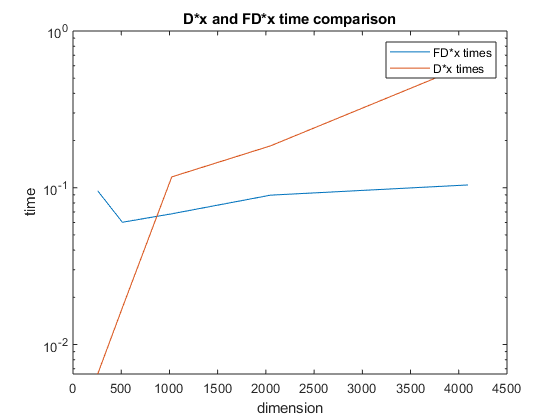As shown for dimensions n above 1024 an actual speedup occurs, the speedup figure below confirms this result. Improving such a speedup and decreasing the dimensions where it occurs is part of the roadmap for future developments of matfaust.
scatter(dims, d_times./fd_times)
legend('speedup')
title("Speedup factor of FD*x relatively to D*x")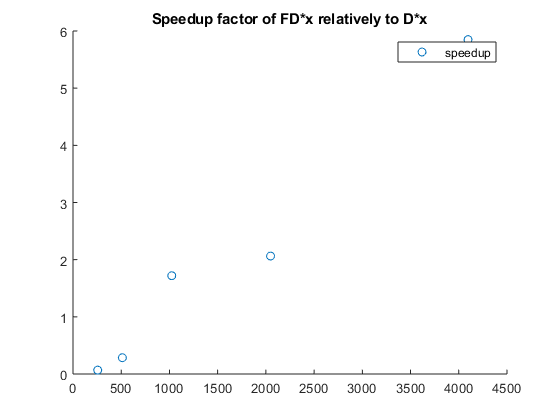The third live script is ending here, I hope you'll be interested in trying yourself to write another algorithm with the FAµST API and maybe discovering any current limitation. Don't hesitate to contact us in that case, we'll appreciate any feedback!